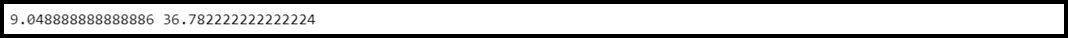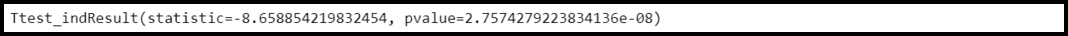Open in App
Not now

# Welch’s t-Test in Python

• Last Updated : 21 Feb, 2022

Welch’s t-Test: Two sample t-Test is used to compare the means of two different independent datasets. But we can apply a Two-Sample T-Test on those data groups that share the same variance. Now to compare two data groups having different variances we use Welch’s t-Test. It is regarded as the parametric equivalent of the Two-Sample T-test.

The user needs to install and import the following libraries to perform Welch’s t-Test in Python:

• scipy
• numpy

Syntax to install all the above packages:

`pip3 install scipy numpy`

Conducting Welch’s t-Test is a step by step process and these are described below,

Step 1: Import the library.

The first step is to import the libraries installed above.

## Python3

 `# Importing libraries ` `import` `scipy.stats as stats ` `import` `numpy as np`

Step 2: Creating data groups.

Let us consider an example, we are given two-sample data, each containing heights of 10 students of a class. We need to check whether two different class students have the same mean height. We can create data groups using numpy.array() method.

## Python3

 `# Creating data groups ` `data_group1 ``=` `np.array([``14``, ``15``, ``15``, ``16``, ``13``, ``8``, ``14``, ` `                        ``17``, ``16``, ``14``, ``19``, ``20``, ``21``, ``15``, ` `                        ``15``]) ` `data_group2 ``=` `np.array([``36``, ``37``, ``44``, ``27``, ``24``, ``28``, ``27``, ` `                        ``39``, ``29``, ``24``, ``37``, ``32``, ``24``, ``26``, ` `                        ``33``])`

Step 3: Check the variance.

Before actually conducting Welch’s t-Test we need to find if the given data groups have the same variance. If the ratio of the larger data groups to the small data group is greater than 4:1 then we can consider that the given data groups have unequal variance. To find the variance of a data group, we can use the below syntax,

Syntax:

print(np.var(data_group))

Here,

data_group: The given data group

## Python3

 `# Python program to display variance  ` `# of data groups ` ` `  `# Import library ` `import` `scipy.stats as stats ` `import` `numpy as np ` ` `  `# Creating data groups ` `data_group1 ``=` `np.array([``14``, ``15``, ``15``, ``16``, ``13``, ``8``, ``14``, ` `                        ``17``, ``16``, ``14``, ``19``, ``20``, ``21``, ``15``, ` `                        ``15``]) ` `data_group2 ``=` `np.array([``36``, ``37``, ``44``, ``27``, ``24``, ``28``, ``27``, ` `                        ``39``, ``29``, ``24``, ``37``, ``32``, ``24``, ``26``, ` `                        ``33``]) ` ` `  `# Print the variance of both data groups ` `print``(np.var(data_group1), np.var(data_group2)) `

Output:variance

Here, the ratio is greater than 4: 1 hence the variance is different. So, we can apply Welch’s t-test.

Step 4: Conducting Welch’s t-Test.

Syntax:

ttest_ind(data_group1, data_group2, equal_var= False)

Here,

data_group1: First data group

data_group2: Second data group

equal_var = “False”: The Welch’s t-test will be conducted by not taking into consideration the equal population variances.

Example:

## Python3

 `# Python program to conduct Welch's t-Test ` ` `  `# Import library ` `import` `scipy.stats as stats ` `import` `numpy as np ` ` `  `# Creating data groups ` `data_group1 ``=` `np.array([``14``, ``15``, ``15``, ``16``, ``13``, ``8``, ``14``, ` `                        ``17``, ``16``, ``14``, ``19``, ``20``, ``21``, ``15``, ` `                        ``15``]) ` `data_group2 ``=` `np.array([``36``, ``37``, ``44``, ``27``, ``24``, ``28``, ``27``, ` `                        ``39``, ``29``, ``24``, ``37``, ``32``, ``24``, ``26``, ` `                        ``33``]) ` ` `  `# Conduct Welch's t-Test and print the result ` `print``(stats.ttest_ind(data_group1, data_group2, equal_var ``=` `False``)) `

Output:Welch’s t-Test

#### Interpretation of the Output:

The test statistic turns out to be -8.658 and the corresponding p-value is 2.757e-08. Here the p-value is less than 0.05 hence we could reject the null hypothesis of the test and the conclusion that the difference between the mean exam score of both types of students is quite significant.

My Personal Notes arrow_drop_up
Related Articles